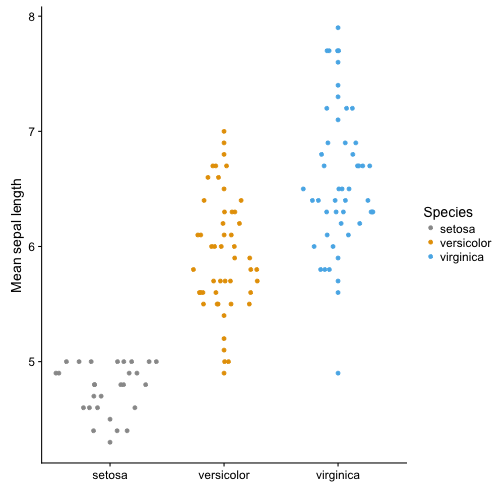# Alternatives to barplots

I reviewed a paper the other day. The data was presented in a barplot and a collegue told me to suggest the authors to use a boxplot or something similar instead. So, I thought I would make some suggestions of alternatives to barplots.

BARPLOTS

Barplots are very commonly used in newspapers or magazins to show numbers. But they are often misused. Barplots should be used to plot count data, e.g. histograms. For plotting any other data, they are less well suited. The problem with barplots is that they hide a lot of useful information and there are better ways to plot your data.

Barplots show a single value (e.g. a mean of many data points) and error bars can be added.

``````# Define colours (color blind palette: http://www.cookbook-r.com/Graphs/Colors_(ggplot2)/)
cbPalette <- c("#999999", "#E69F00", "#56B4E9", "#009E73", "#F0E442", "#0072B2", "#D55E00", "#CC79A7")

# Draw a barplot of mean Septal length of three iris species
iris %>%
group_by(Species) %>%
summarize(n = n(), Mean.Sepal.Length = mean(Sepal.Length), SE = sd(Sepal.Length)/sqrt(n)) %>%
ggplot(aes(y = Mean.Sepal.Length, x = Species, ymin = Mean.Sepal.Length - SE, ymax = Mean.Sepal.Length + SE, fill = Species)) +
geom_bar(stat = "identity") +
geom_errorbar(width = 0.15) +
scale_fill_manual(values = cbPalette) +
labs(x = "", y = "Mean sepal length")
``````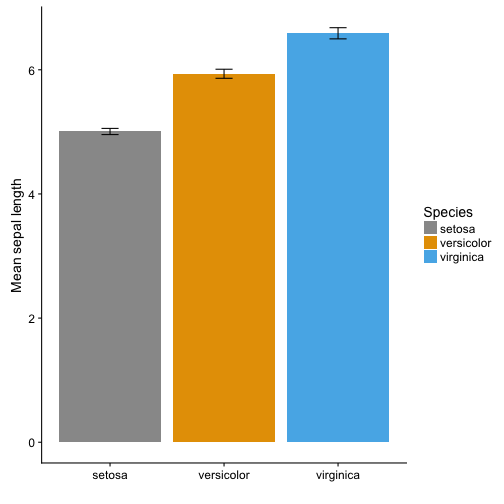BOXPLOT

Boxplots are more informative. They show the median (thick line), first and third quartile (box), wiskers showing the minimum/maximum (for exact definition type ?geom_boxplot) and outliers (points).

``````# Draw a boxplot of mean Septal length of three iris species

g <- ggplot(iris, aes(y = Sepal.Length, x = Species, fill = Species)) +
scale_fill_manual(values = cbPalette) +
labs(x = "", y = "Mean sepal length")
g +   geom_boxplot()
``````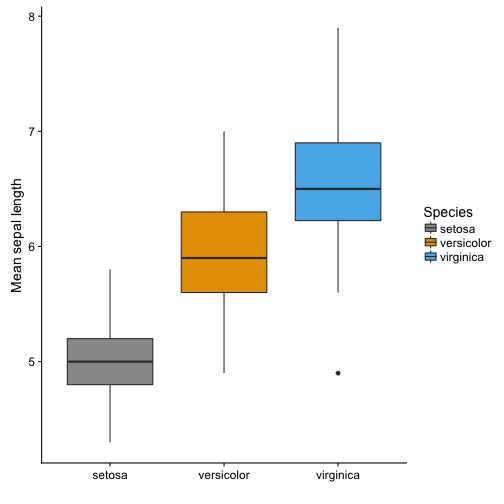In ggplot it is possible to plot several layers on top of each other. In addition to the boxplot it is possible to plot each observation using geom_point() or geom_jitter(). This adds information about the sample size.

``````g +   geom_boxplot() +
geom_jitter(shape = 16, colour = "grey", alpha = 0.5, width = 0.2)
``````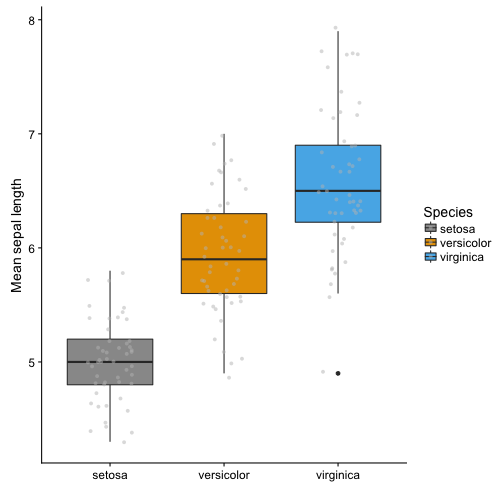VIOLIN PLOT

Violin plots give you even more information about the data. They also show the kernel probability density of the data at different values. It is also possible to show median and the quartiles, like a normal boxplot, use draw_quantiles = c(0.25, 0.5, 0.75). Or you can add a boxplot on top of the violin plot with adding: + geom_boxplot(width = 0.2)

An alternative is to use stat_summary to plot mean and standard deviation insde the violin plot.

``````# Draw violin plot
g + geom_violin(trim = FALSE) +
stat_summary(
fun.data = "mean_sdl",  fun.args = list(mult = 1),
geom = "pointrange", color = "black"
)
``````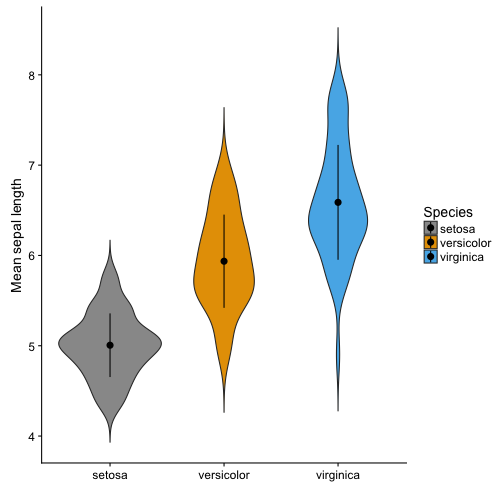SINA PLOT

A sinaplot is useful, becuase is also shows you the sample size of the data. The sample size is usually mentioned somewhere in the text, but it is nice to have it visually presented in the figures. Especially when different groups have different sample sizes.

The sinaplot shows each data point and they are arranged like a violin plot. So you have the sample size, density distribution.

“sinaplot is inspired by the strip chart and the violin plot. By letting the normalized density of points restrict the jitter along the x-axis the plot displays the same contour as a violin plot, but resemble a simple strip chart for small number of data points. In this way the plot conveys information of both the number of data points, the density distribution, outliers and spread in a very simple, comprehensible and condensed format” (https://cran.r-project.org/web/packages/sinaplot/vignettes/SinaPlot.html)

``````library("ggforce")

# removing some observations to get uneven sample size
iris2 <- iris %>%
filter(!(Species == "setosa" & Sepal.Length > 5))

# Sinaplot

ggplot(iris2, aes(y = Sepal.Length, x = Species)) +
labs(x = "", y = "Mean sepal length") +
geom_sina(aes(colour = Species), size = 1.5) +
scale_color_manual(values = cbPalette)
``````